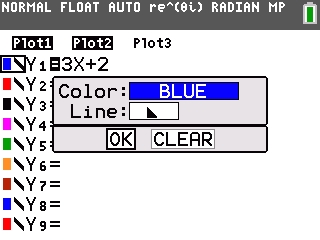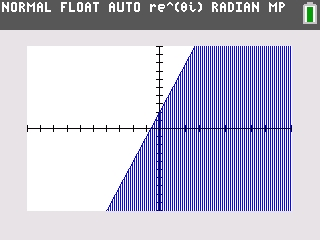# Knowledge Base

## Solution 34916: Graphing Inequalities on a TI-84 Plus C Silver Edition Graphing Calculator.

### How do I graph inequalities on a TI-84 Plus C Silver Edition graphing calculator?

The Y= Editor does not allow an inequality to be directly entered into the calculator. However, the graph styles feature can be utilized to show the solution set for an inequality. The example below will demonstrate how to graph a simple inequality using these features.

Example: Graph y<=3x+2.

On the graphing calculator:

1) Press the [Y=].
2) Press  [X,T,O,n] [+]  on Y1.
3) Press [◄] six times until the symbol to the left of Y1 is flashing.
4) Press [ENTER] [▼] to select the Line area.
5) Press [►] repeatedly until a triangle pointing to the lower part is displayed.
6) Press [▼] [ENTER].
7) This will change the graph style to shade below the function (this style was chosen because the solution set consists of all real numbers below the line of the y= equation).8) Press [GRAPH] to graph the solution set.The Inequality Graphing App can also be used to graph this solution.

Please see the TI-84 Plus C Silver Edition guidebook for additional information.